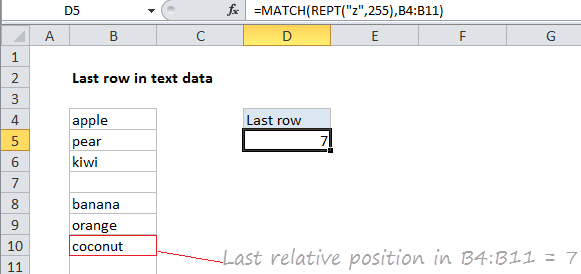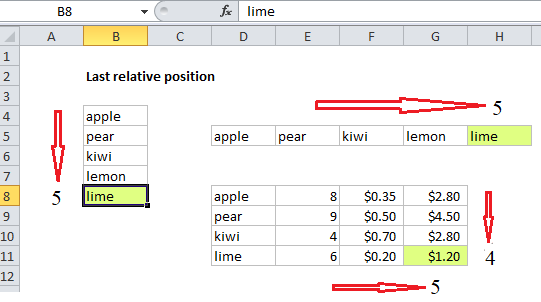## Excel Office

Excel How Tos, Tutorials, Tips & Tricks, Shortcuts

# How to get last row in text data in Excel

To get the last relative position (i.e. last row, last column) for text data (with or without empty cells), you can use the MATCH function. See example below:

## Formula

`=MATCH(bigtext,range)`## Explanation

In the example shown, the formula in E5 is:

`=MATCH(REPT("z",255),B4:B11)`

### How this formula works

This formula uses the MATCH function in approximate match mode to locate the last text value in a range. Approximate match enabled by setting by the 3rd argument in MATCH to 1, or omitting this argument, which defaults to 1.

Also See:   How to calculate next scheduled event in Excel

The lookup value is a so-called “big text” (sometimes abbreviated “bigtext”) which is intentionally a value “bigger” than any value that will appear in the range. When working with text, which sorts alphabetically, this means a text value that will always appear at the end of the alphabetic sort order.

Since this formula matches text, the idea is to construct a lookup value that will never occur in actual text, but will aways be last. To do that, we use the REPT function to repeat the letter “z” 255 times. The number 255 represents the largest number of characters that MATCH allows in a lookup value.

Also See:   How to generate random number weighted probability in Excel

When MATCH can’t find this value, it will “step back” to the last text value in the range, and return the position of that value.

Note: this approach works fine with empty cells in the range, but is not reliable with mixed data that includes both numbers and text.

### Last relative position vs last row number

When building advanced formulas that create dynamic ranges, it’s often necessary to figure out the last location of data in a list. Depending on the data, this could be the last row with data, the last column with data, or the intersection of both. Note: we want the last *relative position* inside a given range, not the row number on the worksheet:

Also See:   Extract most frequently occurring text in Excel